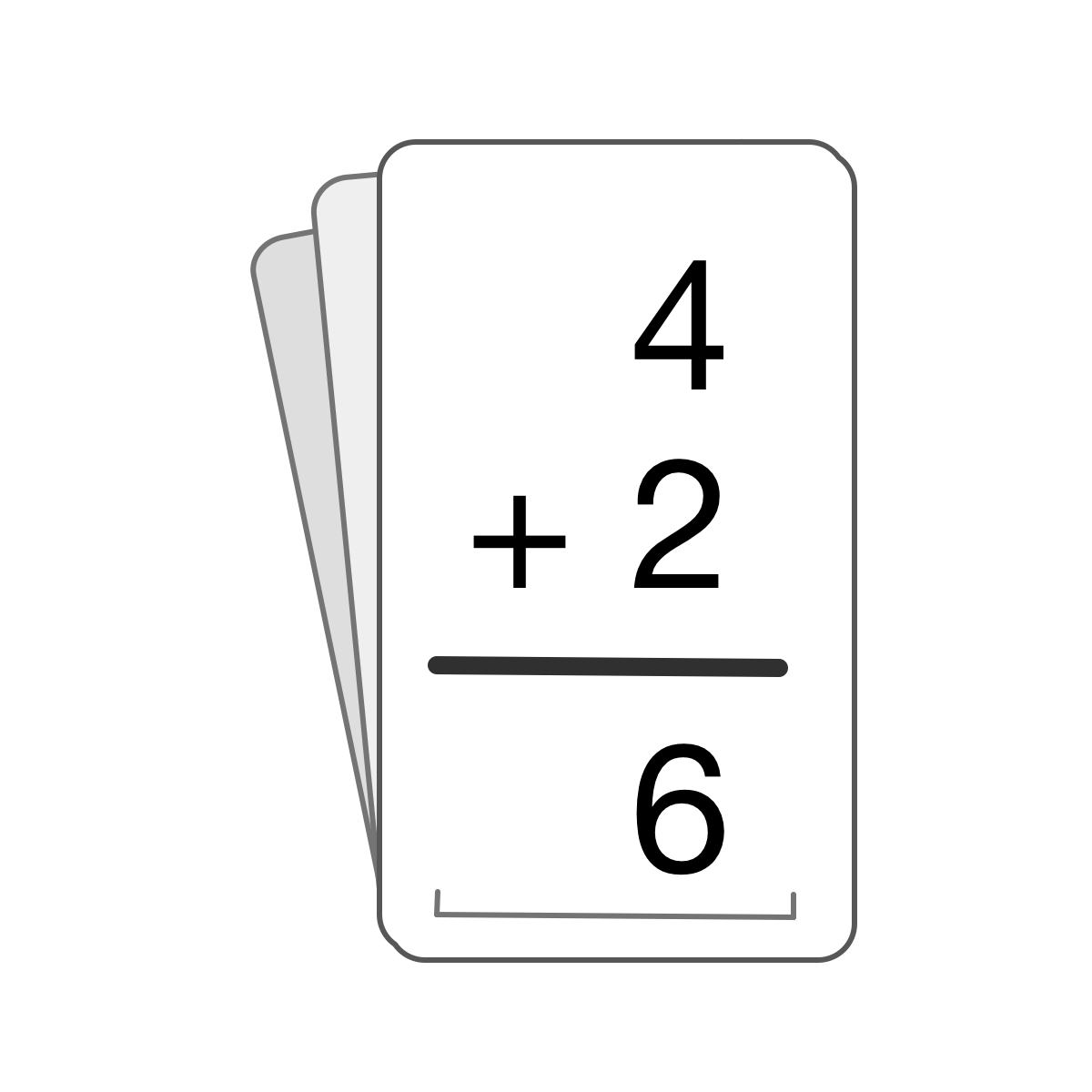# Flash Cards: Math Facts

App for kids to practice math facts; addition, subtraction, multiplication and division
iOS Beta
Education
FULL!

# What to test?

Besides basic  functionality, I added support for Spanish. Would like to have my translations validated.

# App Overview

Flash Cards: Math Facts lets your math learners practice their math facts.

Learners can practice:
-Subtraction
-Multiplication
-Division

With Flash Cards: Math Facts, learners can practice anywhere and anytime.  Quickly configure Flash Cards: Math Facts for a quick set of 10 addition flash cards or create a challenge and configure for a set of division problems.

There are two types of problems.
- Given two numbers, supply the answer.
- Given one number and the answer, supply the 2nd number.

When all flash cards are solved, learners can see the results.  The results are saved so parents can track progress.

Instead of using the same, repeatable paper flash cards Flash Cards: Math Facts can be configured in a multitude of ways.

- Choose a single operation or a combination of all operations.
- Choose how many problems in a set from 1 to 99
- Choose the largest and smallest numbers, all problems will be within this range
- Choose a common number.  That number will appear in all problems.  A great option when practicing your multiplication facts!

While there are a lot of options for Flash Cards: Math Facts, there are a couple rules to help learners.

-The largest number will always appear on top
-Zero can be selected, but it cannot be the top number.
-If a problem is incorrect after 3 tries, learners can skip that problem or keep trying.

A couple additional rules for division.
-Division problems will only be generated with a divisor between the smallest number up to 12.  Choosing zero for division is not an option.

# Screenshots